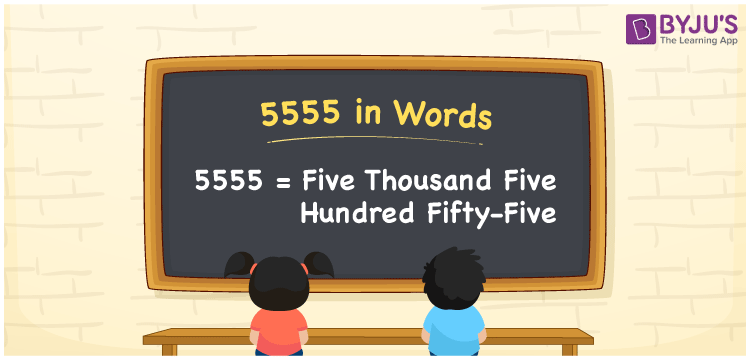# 5555 in Words

5555 in words is given by Five Thousand Five Hundred Fifty-Five. For instance, if you donated Rs. 5555 for the education of blind children, then you can say, “I donated Rupees Five Thousand Five Hundred Fifty-Five for the education of blind children”. 5555 is a cardinal number. The number 5555 is 55 more than the number 5500. It is essential to learn numbers in words to express them in an efficient manner. Let us learn how to write 5555 in words in this article.

 5555 in Words Five Thousand Five Hundred Fifty-Five Five Thousand Five Hundred Fifty-Five in numerical form 5555

## 5555 in English Words

Generally, we use the English alphabet to write numbers in words. Therefore, we can write the number 5555 in English as Five Thousand Five Hundred Fifty-Five.## How to Write 5555 in Words?

Determine the position of each digit in the given number while writing its numerical name. The table provided below shows the place values for the number 5555.

 Thousands Hundreds Tens Ones 5 5 5 5

Therefore, we can write the expanded form as:

5 x Thousand + 5 x Hundred + 5 x Ten + 5 x One

= 5 x 1000 + 5 x 100 + 5 x 10 + 5 x 1

= 5000 + 500 + 50 + 5

= 5555

= Five Thousand Five Hundred Fifty-Five

Hence, 5555 in words is written as Five Thousand Five Hundred Fifty-Five

Interesting way of writing 5555 in words

5 = Five

55 = Fifty-Five

555 = Five Hundred and Fifty-Five

5555 = Five Thousand Five Hundred Fifty-Five

Thus, the word form of the number 5555 is Five Thousand Five Hundred Fifty-Five

5555 is a natural number that precedes 5556 and succeeds 5554

• 5555 in words – Five Thousand Five Hundred Fifty-Five
• Is 5555 an odd number? – Yes
• Is 5555 an even number? – No
• Is 5555 a perfect square number? – No
• Is 5555 a perfect cube number? – No
• Is 5555 a prime number? – No
• Is 5555 a composite number? – Yes

## Frequently Asked Questions on 5555 in Words

Q1

### How to write 5555 in words?

We can write 5555 in words is Five Thousand Five Hundred Fifty-Five.
Q2

### Simplify 5000 + 555, and express in words.

Simplifying 5000 + 555, we get 5555. Therefore, the number 5555 in words is Five Thousand Five Hundred Fifty-Five.
Q3

### Is 5555 an odd number?

Yes, the number 5555 is an odd number.